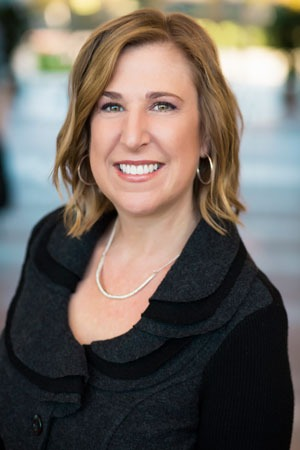# BOARD OF DIRECTORS### Allison Sanford

#### Board Chair### Marie Andel

#### Vice Chair### Robert Brent

#### Vice Chair### Wendy Smit

#### Secretary``` ``` ``` `````` ``` ``` `````` ``` ``` `````` ``` ``` `````` ``` ``` `````` ``` ``` `````` ``` ``` ```

### Jennifer Bice

``` ``` ``` ``` ``` ``` ``` ``` ``` ``` ``` ``` ``` ``` ``` ``` ``` ``` ``` ``` ``` ``` ``` ``` ``` ``` ``` `````` ``` ``` `````` ``` ``` `````` ``` ``` `````` ``` ``` `````` ``` ``` `````` ``` ``` `````` ``` ``` `````` ``` ``` ```

### Simon Blattner

``` ``` ``` ``` ``` ``` ``` ``` ``` ``` ``` ``` ``` ``` ``` ``` ``` ``` ``` ``` ``` ``` ``` ``` ``` ``` ``` ``` ``` ``` ``` `````` ``` ``` `````` ``` ``` `````` ``` ``` `````` ``` ``` `````` ``` ``` `````` ``` ``` `````` ``` ``` `````` ``` ``` ```

### Clay Clement

``` ``` ``` ``` ``` ``` ``` ``` ``` ``` ``` ``` ``` ``` ``` ``` ``` ``` ``` ``` ``` ``` ``` ``` ``` ``` ``` ``` ``` ``` ``` `````` ``` ``` `````` ``` ``` `````` ``` ``` `````` ``` ``` `````` ``` ``` `````` ``` ``` `````` ``` ``` `````` `````` ``` ``` `````` ``` ``` ```

### Scott Hafner

``` ``` ``` ``` ``` ``` ``` ``` ``` `````` ``` ``` `````` ``` ``` ``` ``` ``` ``` ``` ``` ``` ``` ``` ``` ``` ``` ``` ``` ``` ``` ``` ``` ``` ``` ``` ``` `````` ``` ``` `````` ``` ``` `````` ``` ``` `````` ``` ``` `````` ``` ``` `````` ``` ``` `````` ``` ``` `````` `````` ``` ``` `````` ``` ``` ```

### Duncan Higgons

``` ``` ``` ``` ``` ``` ``` ``` ``` `````` ``` ``` `````` ``` ``` ``` ``` ``` ``` ``` ``` ``` ``` ``` ``` ``` ``` ``` ``` ``` ``` ``` ``` ``` ``` ``` ``` `````` ``` ``` `````` ``` ``` `````` ``` ``` `````` ``` ``` `````` ``` ``` `````` ``` ``` `````` `````` ``` ``` `````` ``` ``` `````` ``` ``` ```

### Steven Hightower

``` ``` ``` ``` ``` ``` ``` ``` ``` ``` ``` ``` ``` `````` ``` ``` `````` ``` ``` ``` ``` ``` ``` ``` ``` ``` ``` ``` ``` ``` ``` ``` ``` ``` ``` ``` ``` `````` ``` ``` `````` ``` ``` `````` ``` ``` `````` ``` ``` `````` ``` ``` `````` ``` ``` `````` ``` ``` `````` ``` ``` ```

### Philip Jensen

``` ``` ``` ``` ``` ``` ``` ``` ``` ``` ``` ``` ``` ``` ``` ``` ``` ``` ``` ``` ``` ``` ``` ``` ``` ``` ``` ``` ``` ``` ``` `````` ``` ``` `````` ``` ``` `````` ``` ``` `````` ``` ``` `````` ``` ``` `````` ``` ``` `````` ``` ``` `````` ``` ``` ```

### Perry Karsen

``` ```

``` ``` ``` ``` ``` ``` ``` ``` ``` ``` ``` ``` ``` ``` ``` ``` ``` ``` ``` ``` ``` ``` ``` ``` ``` ``` ``` ``` ``` ``` ``` ```### Nancy Otto### Tim Portwood### Ellie Rilla### Judy Scotchmoor### Peter Van Alyea### Denny Van Ness### Lewis Warren